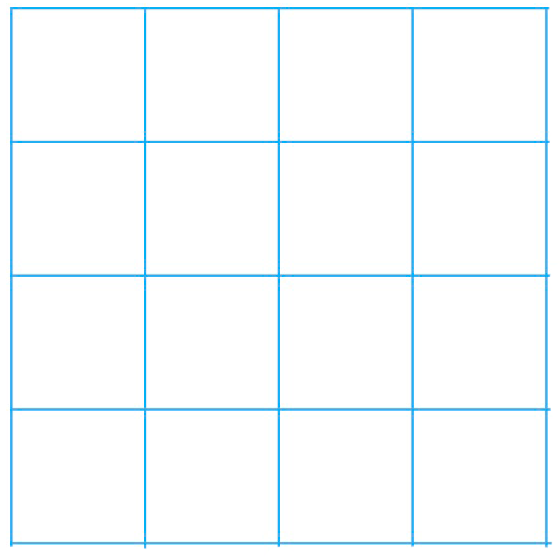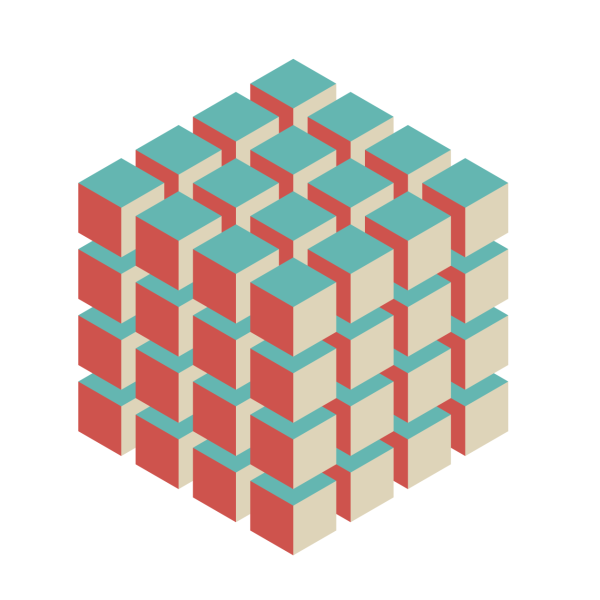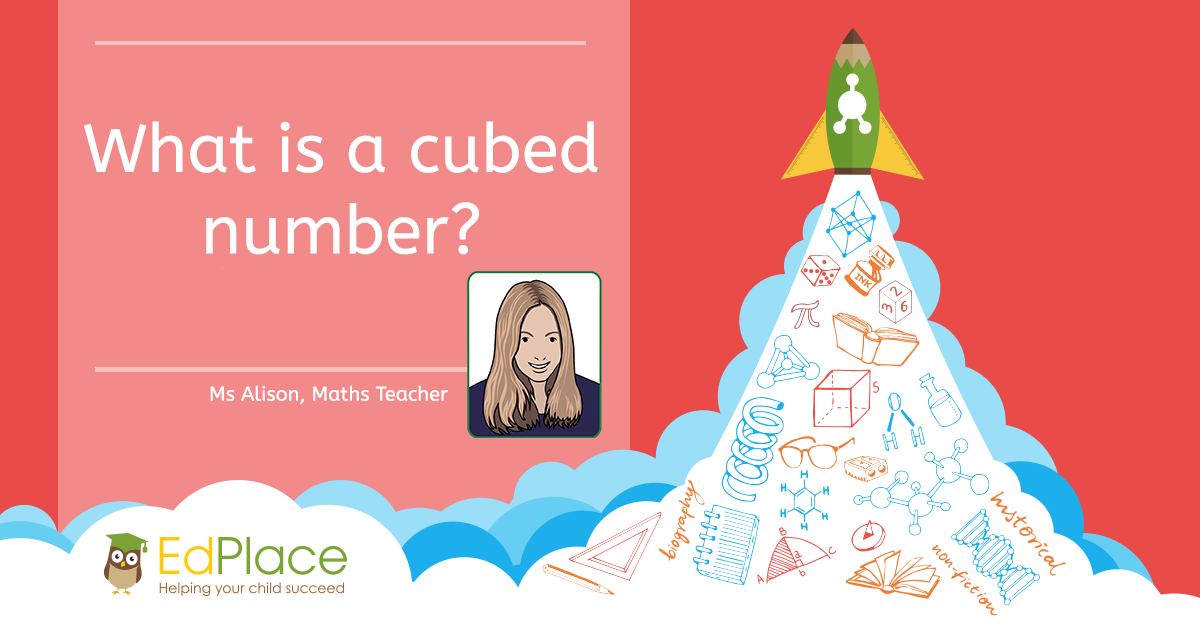# What is a cubed number?

When you multiply a whole number (not a fraction) by itself, and then by itself again the result is a cube number. For example 3 x 3 x 3 = 27.

An easy way to write 3 cubed is 33. This means three multiplied by itself three times.

The easiest way to do this calculation is to do the first multiplication (3x3) and then to multiply your answer by the same number you started with; 3 x 3 x 3 = 9 x 3 = 27.

# Learning Cube Numbers

Cube numbers can be a little bit more confusing than squared numbers, simply because of the extra multiplication. Essentially, you are calculating a 3D shape instead of a flat one.

Here is a flat (or 2D) 4 x 4 square:To calculate the number of blocks (the squared number) we would simply multiply 4 x 4 or 42equalling 24.

Here is a 3D 4 x 4 cube:To calculate the number of blocks (the cubed number) this time we would multiply 4 x 10 x 4 or 43 equalling 64.

In KS2, you won’t need to learn cube numbers off by heart, but you will have to have a basic understanding of what they are, and how to calculate them. Often children will be given a pattern of numbers, such as lower end cube numbers and may be asked to try to work out the pattern.

Here is a list of cubed numbers up to 12x12:

 0 Cubed = 03 = 0 × 0 x 0 = 0 1 Cubed = 13 = 1 × 1 x 1 = 1 2 Cubed = 23 = 2 × 2 x 2 = 8 3 Cubed = 33 = 3 × 3 x 3 = 27 4 Cubed = 43 = 4 × 4 x 4 = 64 5 Cubed = 53 = 5 × 5 x 5 = 125 6 Cubed = 63 = 6 × 6 x 6 = 216 7 Cubed = 73 = 7 × 7 x 7 = 343 8 Cubed = 83 = 8 × 8 x 8 = 512 9 Cubed = 93 = 9 × 9 x 9 = 729 10 Cubed = 103 = 10 × 10 x 10 = 1,000 11 Cubed = 113 = 11 × 11 x 11 = 1,331 12 Cubed = 123 = 12 × 12 x 12 = 1,728

# Finding the Cube of a Negative Number.

The cube of a negative number will always be negative, just like the cube of a positive number will always be positive.

For example; -53 = -5 x -5 x- -5 = (25 x -5) = -125.

# Finding the Cube of a Decimal.

Just like whole numbers (integers), it’s easy to cube a decimal number too. Don’t worry though, you won’t need to memorise these in key stage 2 (or probably even work them out)!

 1.23 Cubed = 1.233 = 1.23 × 1.23 x 1.23 = 1.86087 2.56 Cubed = 2.563 = 2.56 × 2.56 x 2.56 = 16.7772

# Worksheets and Practice

Here are some worksheets aimed specifically at getting to grips with cube numbers and practising your skills.

Year 6 – Drawing dice dots on net cubes

Year 8 – Cube numbers and cube roots

# Further Learning

If cube numbers and puzzles are your thing and you really want to give yourself a challenge, why not look at the BBC Bitesize website or try some of the puzzles and problems set by the NRich team at the University of Cambridge?

https://nrich.maths.org/public/leg.php?code=-308

http://www.bbc.co.uk/guides/z2ndsrd

## Wait, don’t go! Keep Learning with EdPlace###### AUTHOR, MS ALISON – MATHS TEACHER.